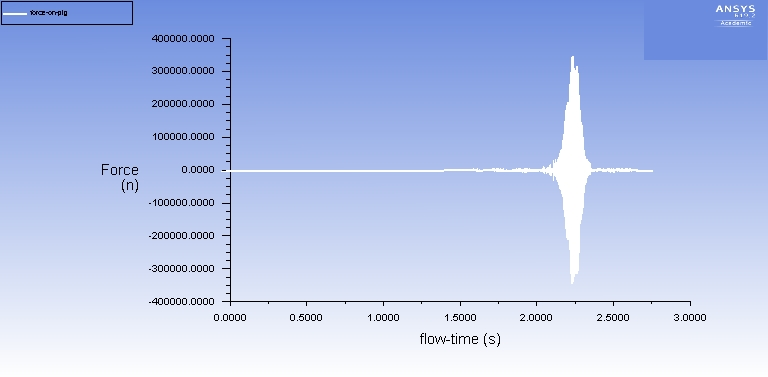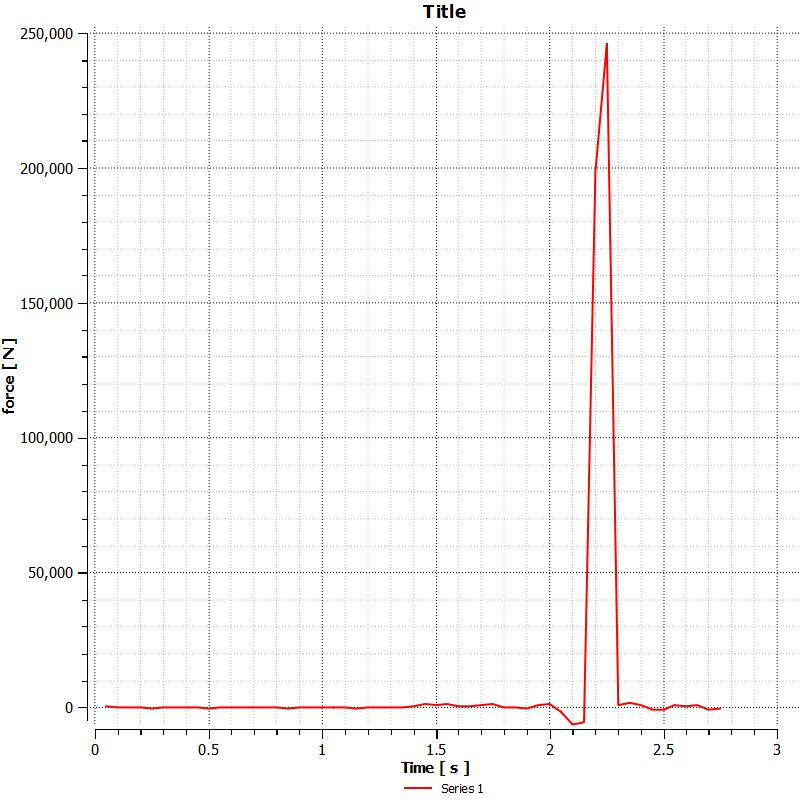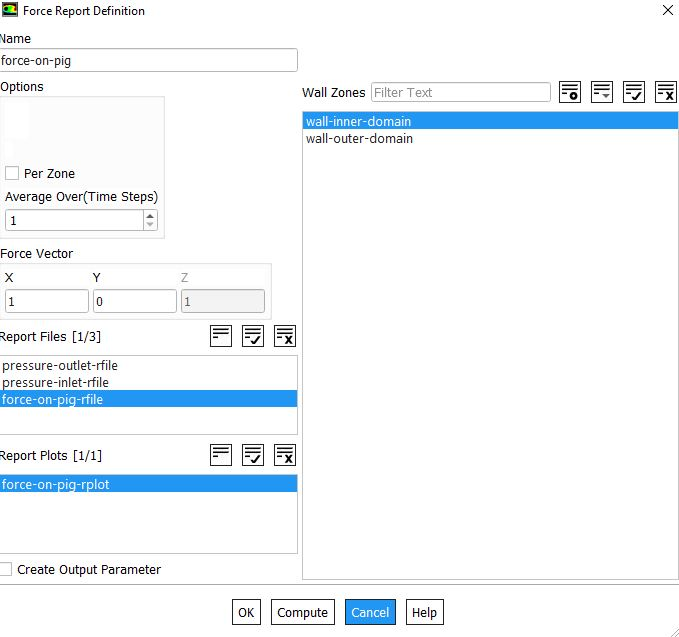# Graph meaningBoth are results for the same simulation. first one taken from fluent while simulation running second one after post processing.

what does the first graph mean by showing negative numbers?

• edited November 2018

Can you post the monitor you're using?

• edited November 2018• edited November 2018

OK, the force plot has a vector in positive x. If the forces act in the other direction you'll get a negative value. Is the second plot the force magnitude in CFD Post?